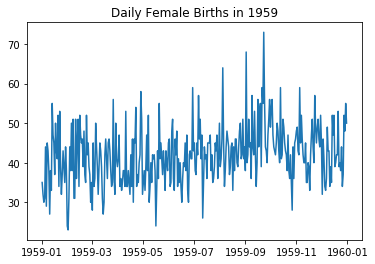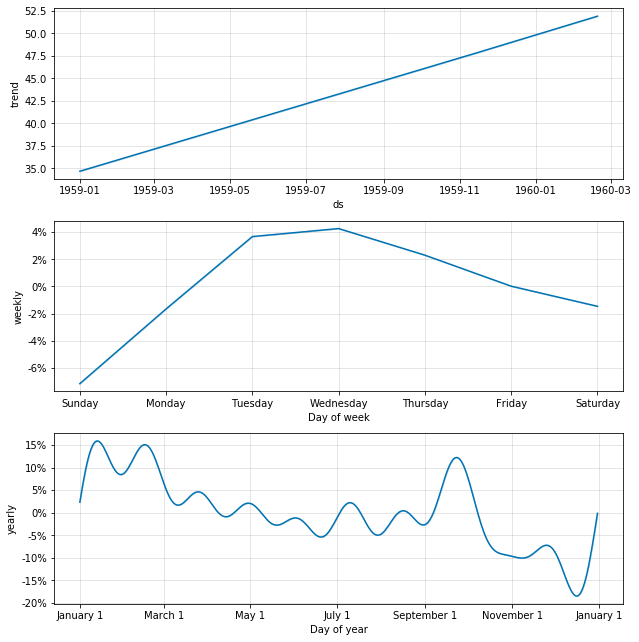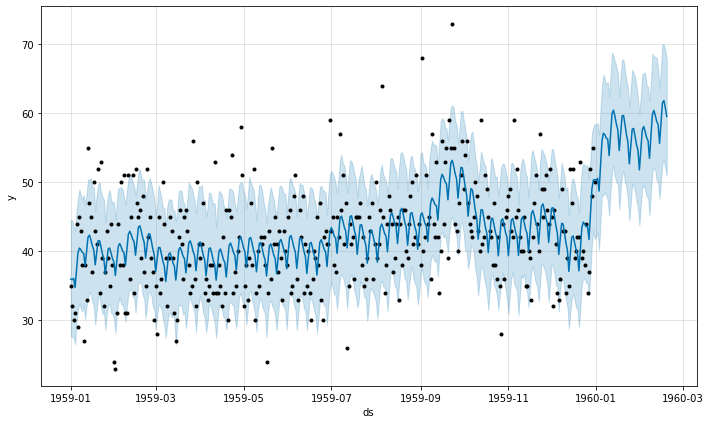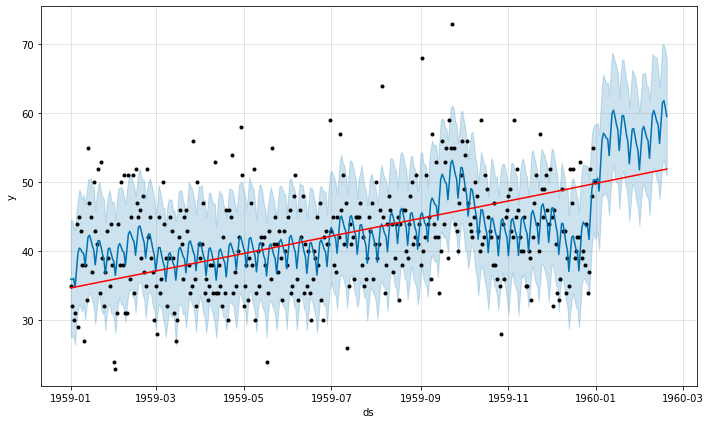# Time Series Forecasting for Daily Births Dataset by Prophet

## Goal¶

This post aims to introduce how to forecast the time series data for daily births dataset using Prophet.

Prohpeht Forecasting Model

$$y (y) = g(t) + s(t) + h(t) + \epsilon_t$$
• $g(t)$: a growth term, which is a trend curve
• $s(t)$: a seasonality term, which periodically changes
• $h(t)$: a holiday term, which indicates irregular events (given by users)
• $\epsilon_t$: an error term

Reference

## Libraries¶

In :
import pandas as pd
import numpy as np
import fbprophet
import warnings
import matplotlib.pyplot as plt
%matplotlib inline


## Load a female daily births dataset¶

In :
url = "https://raw.githubusercontent.com/jbrownlee/Datasets/master/daily-total-female-births.csv"
df.columns = ['ds', 'y']

Out:
ds y
0 1959-01-01 35
1 1959-01-02 32
2 1959-01-03 30
3 1959-01-04 31
4 1959-01-05 44

### Visualize the raw data¶

In :
plt.plot(df['ds'], df['y']);
plt.title('Daily Female Births in 1959');## Create a Prophet instance¶

In :
with warnings.catch_warnings():
warnings.simplefilter("ignore")
m = fbprophet.Prophet(yearly_seasonality=True, daily_seasonality=False,
changepoint_range=0.9,
changepoint_prior_scale=0.5,
seasonality_mode='multiplicative')
m.fit(df);

In :
future = m.make_future_dataframe(periods=50, freq='d')
forecast = m.predict(future)


## Visualize¶

### By Component¶

In :
m.plot_components(forecast);### Plot raw data with forecast¶

In :
m.plot(forecast);## Change point detection¶

No change is detected.

In :
fig = m.plot(forecast)